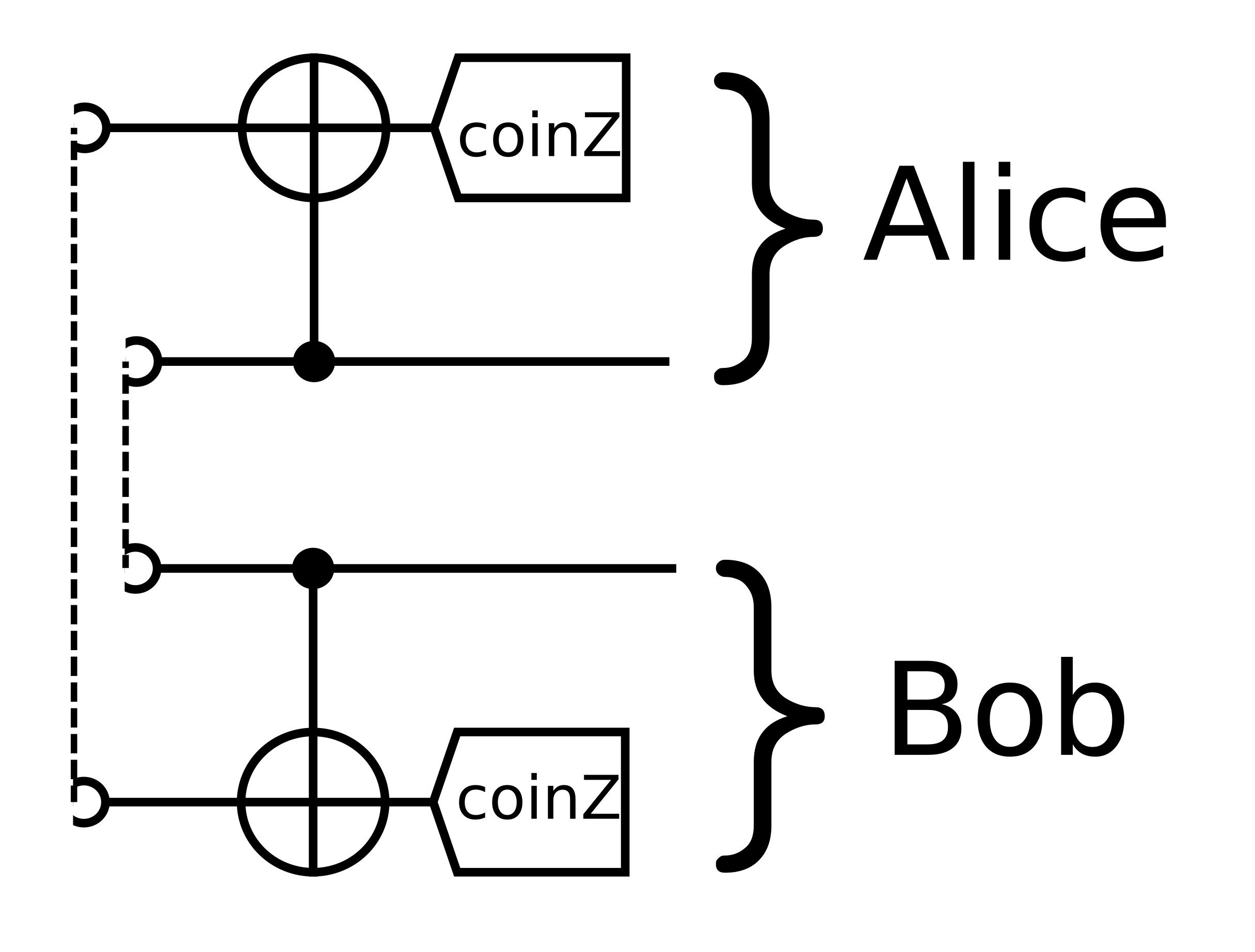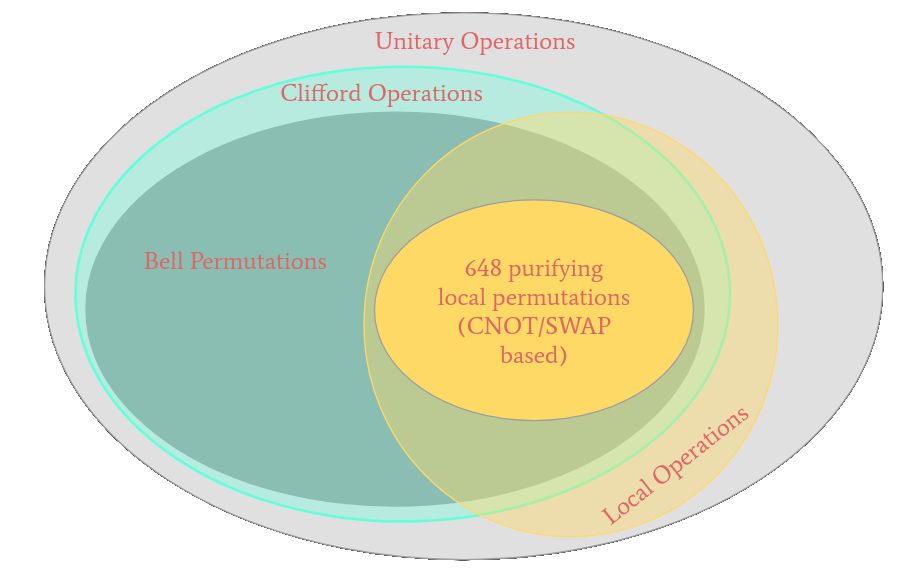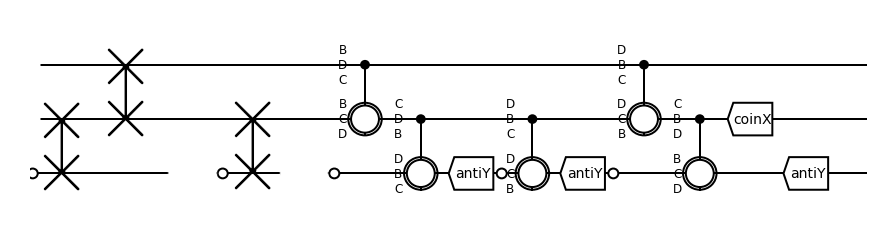# Noisy Entanglement (on Color Centers)

Stefan Krastanov | MIT

## Entanglement

They can be entangled! \begin{aligned} A=|\phi_{+}\rangle=\frac{|00\rangle+|11\rangle}{\sqrt{2}}\\ B=|\psi_{-}\rangle=\frac{|01\rangle-|10\rangle}{\sqrt{2}}\\ C=|\psi_{+}\rangle=\frac{|01\rangle+|10\rangle}{\sqrt{2}}\\ D=|\phi_{-}\rangle=\frac{|00\rangle-|11\rangle}{\sqrt{2}} \end{aligned}
They can be entangled! \begin{aligned} A=|\phi_{+}\rangle=\frac{|00\rangle+|11\rangle}{\sqrt{2}}\\ B=|\psi_{-}\rangle=\frac{|01\rangle-|10\rangle}{\sqrt{2}}\\ C=|\psi_{+}\rangle=\frac{|01\rangle+|10\rangle}{\sqrt{2}}\\ D=|\phi_{-}\rangle=\frac{|00\rangle-|11\rangle}{\sqrt{2}} \end{aligned}
1. Krastanov et al.
Art installation on Non-contextual Hidden Variable theories

## Entanglement with Color Centers

### Entanglement Protocols

Inherently low probability of success (DLCZ-like)

Full excitation and path erasure (Barret-Kok)

Single-photon reflection

Atom-photon gates (Duan-Kimble)

### Atom-photon gates (Duan-Kimble)

Rate only classical light no optical excitation
DLCZ $$\eta (1-F)$$
BK $$\eta^2$$
reflection $$\eta^2$$

You also need a color center that does not suffer from spectral diffusion or charge state instabilities.

If you end up with significant optical excitation, you also need a way to turn off the hyperfine coupling to the (nuclear) memory.

## First Steps to Entanglement Purification

### They can be noisy!

Desired:

\begin{aligned} A=\frac{|00\rangle+|11\rangle}{\sqrt{2}}\\ \end{aligned}

The hardware generated:

90% chance for \begin{aligned} A=\frac{|00\rangle+|11\rangle}{\sqrt{2}}\\ \end{aligned} 10% chance for a bit flip on Bob's qubit \begin{aligned} C=\frac{|01\rangle+|10\rangle}{\sqrt{2}}\\ \end{aligned}

### Purification of entanglement

\begin{aligned} A=|\phi_{+}\rangle=\frac{|00\rangle+|11\rangle}{\sqrt{2}}\\ B=|\psi_{-}\rangle=\frac{|01\rangle-|10\rangle}{\sqrt{2}}\\ C=|\psi_{+}\rangle=\frac{|01\rangle+|10\rangle}{\sqrt{2}}\\ D=|\phi_{-}\rangle=\frac{|00\rangle-|11\rangle}{\sqrt{2}} \end{aligned}
\begin{aligned} A\propto|00\rangle+|11\rangle\\ B\propto|01\rangle-|10\rangle\\ C\propto|01\rangle+|10\rangle\\ D\propto|00\rangle-|11\rangle \end{aligned}\begin{aligned} A\propto|00\rangle+|11\rangle\\ B\propto|01\rangle-|10\rangle\\ C\propto|01\rangle+|10\rangle\\ D\propto|00\rangle-|11\rangle \end{aligned}\begin{aligned} A\propto|00\rangle+|11\rangle\\ B\propto|01\rangle-|10\rangle\\ C\propto|01\rangle+|10\rangle\\ D\propto|00\rangle-|11\rangle \end{aligned}... How are we going to find the "good" permutation?What if we want multi-sacrifice circuit?

### Discrete Optimization: Evolutionary Algorithm

1. Krastanov et al.
Optimized Entanglement Purification
1. Krastanov et al.
Optimized Entanglement Purification
1. Krastanov et al.
Optimized Entanglement Purification

### Circuit ConstraintsIn some hardware types only one of the qubits can establish entanglement.

### Ongoing projects and useful tools

Faster purificaiton simulators¹

n-to-k purification circuits²

Rigorous theory bounds on purification performance³

QuantumClifford.jl and QuantumSavory.jl